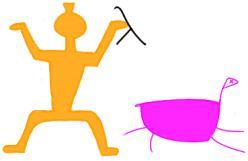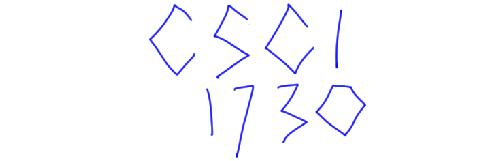Complete this assignment with the same team you worked with for Continuations (Written). You and your partner must each understand the answers to all the problems, so don't just split up the work.

### Type Checker

In this assignment, you will work with a typed language that includes numbers, booleans, conditionals, functions, and numeric lists. The concrete syntax for the language is given by the following BNF grammars:

```   <expr> ::= <num>
| true
| false
| {+ <expr> <expr>}
| {- <expr> <expr>}
| {* <expr> <expr>}
| {/ <expr> <expr>}
| {zero? <expr>}
| {bif <expr> <expr> <expr>}

| <id>
| {with {<id> : <type> <expr>} <expr>}
| {fun {<id> : <type>} : <type> <expr>}
| {<expr> <expr>}

| nempty
| {ncons <expr> <expr>}
| {nempty? <expr>}
| {nfirst <expr>}
| {nrest <expr>}

<type> ::= number
| boolean
| nlist
| (<type> -> <type>)

```
where an `<id>` is not ```true, false, :, +, -, *, /, zero?, bif, with, fun, nempty, ncons, nempty?, nfirst,``` or `nrest`.

In the surface syntax for types, base types are represented by symbols, and the arrow type by a Scheme list of three elements: the type of the argument, the symbol `->`, and the type of the result.

You have not implemented some of these constructs yet, but they should be familiar:

• `zero?` consumes a number, and returns `true` if it is `0`, `false` otherwise
• the test expression of `bif` ("boolean if") must evaluate to `true` or `false`
• `ncons` consumes a number and a numeric list, and produces a numeric list

### Design and Implement the Type Checker

Your type checker must be written in PLAI Scheme. We provide a template to help you get started. The template has `define-type` definitions for the abstract syntax of the language and its types. Do not change these definitions.

1. Define the function `parse`, which consumes the concrete representation of a program, and returns its abstract syntax tree. To be precise,

`parse :: S-exp -> Expr`
You may assume that s-expression provided to parse conforms to the grammar.

2. Write down type judgments for the five numeric list constructs: `nempty`, `ncons`, `nempty?`, `nfirst`, and `nrest`. Turn in your work as a PDF (preferable) or text file (less preferable) with your code.

3. Implement the function `type-of`, which consumes the abstract representation of a program (i.e. the result of `parse`) If the program has no type errors, `type-of` returns the type of the program, using the names of the types given in the grammar above. To be precise,

`type-of :: Expr -> Type`
However, if the program does have a type error, `type-of` invokes `error` with an appropriate error message. For example:
`(type-of (parse '{+ 1 2}))`
should produce `(t-num)`, while:
`(type-of (parse '{3 4}))`
should call `error` with some string, e.g. "Number is not a function".

### Scheme's `(match ...)` Syntax

One last reminder about Scheme's `(match ...)` syntax. Use it! Love it! As always, it is not required (but at this point highly encouraged for your own sanity).

### Handin

Turn in two files:

1. `typecheck.ss`, your program.
2. `judgments.pdf`, your type judgments.